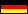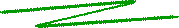The Carmichael function λ(n)The Carmichael function λ is a number theoretic function closely related to the Euler function φ(n). Just like φ, λ has a deep connection to prime numbers and to the order of integers. The name is due to the U.S. mathematician Robert D. Carmichael (1879-1967) (see also here).

The definition of the Carmichael function λ: NN is pretty complicated: If the prime factorization of n is given by n = p1a1 ... pkak, we have λ(n) = lcm[λ(p1a1), ..., λ(pkak)], where

 λ(piai) = { 2ai - 2 if pi = 2 and ai > 2, piai - 1 (pi - 1) otherwise.

(lcm = least common multiple). An example may shed light on this formula: Let be n = 12, with its prime factorization 12 = 22·3; then λ(12) = lcm[λ(22), λ(3)], where λ(22) = 1 and λ(3) = 2, i.e. λ(12) = 2. With appropriate effort one computes the following table of values for the first positive integers (also listed the corresponding values of the Euler totient function φ):

 n λ(n) φ(n) 1 2 3 4 5 6 7 8 9 10 11 12 13 14 15 1 1 2 2 4 2 6 2 6 4 10 2 12 6 4 1 1 2 2 4 2 6 4 6 4 10 4 12 6 8

One of the properties of the Carmichael function, anticipated by the table, can be generalized to an arbitrary integer n: The Carmichael function value λ(n) divides the Euler function value φ(n), in symbols λ(n) | φ(n). For a prime p the Carmichael function values are comparably easy to compute:

λ(p) = p - 1       (p prime).

(Note that in this case λ(p)=φ(p).) For instance, we have λ(7) = 6, or λ(13) = 12. If n is the product of two primes p und q, n=pq, we have λ(pq) = lcm(p-1, q-1). Example: λ(15) = lcm(3-1, 5-1) = 4. Furthermore, we have the following important theorem.

Carmichael's theorem. For two positive relatively prime integers m, n we have

mλ(n) = 1 mod n.

Given a fixed n, λ(n) is the smallest exponent with this property for all m ∈ IN.

You can start an Appletcomputing the Euler and die Carmichael function values.

### References

 © de Vries 2002